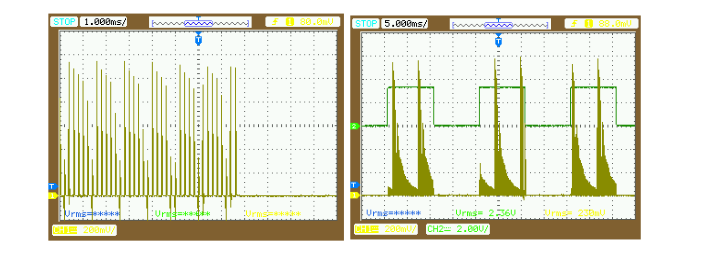# Experiment 1: Single Phase Half Wave Controlled Rectifier with R – Load for Positive Half Cycle

## Objective

To Construct Single Phase Half Wave Controlled Rectifier with R-Load for Positive Half Cycle using SCR and TMS320F28027F.

## Circuit Diagram for Rectifier with R – Load for Positive Half CycleFig 5.3) Circuit Diagram for Single Phase Half Wave Controlled Rectifier with R-LOAD for Positive Half Cycle

## Connection Diagram forRectifier with R – LoadFig 5.4) Connection Diagram for Single Phase Half Wave Controlled Rectifier with R-LOAD for Positive Half Cycle

## Procedure of Single Phase Half Wave Controlled Rectifier for Positive Cycle for R-LOAD

1.Power converter circuit has to be designed  and simulation is verified in Matlab as shown in figure below.

## 3.Simulation Waveforms

4.    After completion of simulation verification, Firing pulse generation circuit has to be detached to generate code for LaunchPad TMS320F28027F.

5.    Now follow the steps in CONFIGURATION SETTINGS TO INTERFACE TMS320F28027F WITH MATLAB.

6.    As shown below, use the Firing pulse generating section alone by replacing the Input and Output ports with GPIO Blocks.

7.    Code Generation for TMS320F28027F LaunchPad  using MatlabFig 5.7) Code Generation using Matlab for Single Phase Half Wave Controlled Rectifier with R-LOAD for Positive Half Cycle

8.    Verify all power to the Trainer Kit is in OFF status.

9.    Place the LaunchPad TMS320F28027F on the board slot as shown in Fig 2.5

10. Power up the LaunchPad using the USB cable connected with the PC/Laptop

11. Follow the steps in PROGRAMMING THE LAUNCHPAD TMS320F28027F USING CODE COMPOSER STUDIO.

12. After programming, LaunchPad is ready to Generate the Pulse. Press reset once.

13. Now connect the Isolation Transformer unit through 14pin cable to the board as Shown in Fig 2.11.

14. Now verify the power switch on the board is in OFF Status and power ON the Isolation Transformer Unit. Compare the ZCD and the generated Pulse from LaunchPad using Oscilloscope.

15.Below Screenshot shows theComparison of generated pulse with the ZCD.

16.  Now verify the pulses across (G1, K1).Fig 5.10) Test points of G1, and K1 to verify in DSO

17. Below Screenshot shows thePulses applied to SCR for TriggeringFig 5.11) Waveform of the pulses applied to SCR captured in DSO

18.  Now connect the Power Circuit as per the Circuit Diagram shown in (Fig: 5.3) or as per the Connection Diagram shown in (Fig:5.4). Verify the connection .

19.  Check the Fuse F1 is proper with (230V/1A) rating.

20.  Now switch ON the Main Power Switch on the Trainer Kit.

21.  Analyze the Output waveforms and current waveforms for various firing angle by pressing the Key (S3) in LaunchPad TMS320F28027F and tabulate the results.

22.  For waveforms in DSO

## 23.  Hardware Output waveformsFig 5.13) Hardware Output model waveforms for Single Phase Half Wave Controlled Rectifier with R-LOAD for Positive Half Cycle

Details gathered

TON = SCR ON time from Output voltage waveform using DSO in ms.

TOFF = SCR OFF time from Output voltage waveform using DSO in ms.

Alpha α = Calculated value using formula in degree.

Vo = Measured Output Voltage using Multimeter and Calculated Output voltage in Volts.

Vpk = Input AC voltage in Volts

## TabulationTable 5.1) Tabulation for Single Phase Half Wave Controlled Rectifier for Positive Half Cycle for R-LOAD:

## Model Calculations

2.Output Voltage

Vo=(Vpk/2p)*(1+cosa)

Where,

Vpk= input ac voltage in volt.

a= firing angle in degree

(Eg.:)

## Conclusion

Thus Single Phase Half Wave Controlled Rectifier with R-Load for Positive Half Cycle using TMS320F28027F was done successfully, Output waveforms and Input waveforms for various firing angle was analyzed and verified.

☞The below screenshot show ,How to configure an constant firing angle.